# Physics - Electromagnetism - Ampere's law and Applications## Introduction

Hello it's a me again Drifter Programming! Today we continue with Electromagnetism to get into the Ampere's law and Applications of this law! So, without further do, let's get straight into it!

## Ampere's law

In the previous posts we used the Biot-Savart law which is: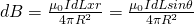An alternative expression of the relation between the magnetic field and the current that produces it is Ampere's law, named in honour of Andre-Marie Ampere! The formal definition states that: "the line integral of the magnetic field around an arbitrarily chosen path is proportional to the net electric current enclosed by the path". Afterwards Maxwell (famous for the Maxwell equations) extended this law to include magnetic fields that arise without current by other causes. This means that Ampere-Maxwell's law is one of the four Maxwell equations!

So why do we need this "other" law? By that I mean why is Biot-Savart's law not enough? Well it's simple. You might remember that Gauss's law gave us results much faster and easier then Coulomb's law. The same way Biot-Savart's law can be applied to any configuration of magnetic fields due to current-carrying conductors/wires, but mostly requires a complicated sum of infinitesimal current elements. So, think of Ampere's law as the analogous of Gauss's law in Electrostatics! Gauss's law helped us when calculating electric fields, cause it allowed symmetry. Ampere's law determines the magnetic field of symmetric systems of current elements (can you see the similarity?). Gauss's law gave us an relation between the regional charge distribution Q and electric field E summed over a surface (Gaussian surface) in the form of electric flux Φ. For Ampere's law we consider paths of current elements or the magnetic field over an entire path. Electric flux is computed over a Gaussian surface. To sum the magnetic field over a closed path we create a so-called Amperian loop. We also don't consider the total charge enclosed, but the total current enclosed by an Amperian loop. Being a loop an Amperian loops needs to be orientated. For that we are using the right hand "curl" rule.

The integral form of the law is: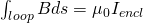And so we calculate a line, closed loop integral of the magnetic field B inside of which a total current Iencl is enclosed. Note that the choice of the Amperian loop is very important, the same way as Gaussian surfaces where important in Gauss's law! To use this equation you can use any closed path that you want and it's very important that this path is closed!

Because we sum/add up we sometimes also write it as an actual sum: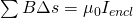From the equations we can see that:

• Increasing the current flowing through a closed path, increased the total magnetic field
• By knowing the magnetic field we can add it up around the path and calculate the current flowing through this closed path.
• Integral(Bdl) = 0 doesn't necessarily mean that the magnetic field B is zero, but only that the current flow is zero!

The extended version contains the concept of displacement current that we will cover in the following up articles and makes the equation look far more complicated!

## Applications

Ampere's law has many practical applications. The main usage is of course calculating the magnetic field generated by an electric current. This is useful in electromagnets, motors, generators, transforms etc. In calculations that could be done using Biot-Savart's law, Ampere's law simplifies the calculation process by using a certain symmetry. Let's get into some calculations!

### Straight wire

The magnetic field "circles" around the wire, which means that we choose an circular Amperian loop of radius r centered at the wire (consider that the wire has no thickness). The magnetic field is constant and equal at all the points, which means that Bds is also constant. By enclosing it in the total circumference from 0 to 2πr the integral gives us 2πr and so we finally have:which is exactly the same that we calculated using Biot-Savart's law!

### Cylindrical conductor / Thick wire

Let's consider the same wire as before, but now with thickness! The outer radius of the wire is R and the current is distributed uniformly along the wire. What is the magnetic field now if the total current is I again?

We again choose a circular Amperian loop of radius r, centered this time at the axis of the wire. If r > R we are outside of the wire, which means that we get the same results as before for a standard thin wire. So, let's say that r <= R, which takes is in the "inside" of the wire. Because the current is uniformly distributed the total enclosed current now is: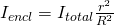And so Ampere's law gives us:The current increases quadratically with r (r^2), whilst the magnetic field increases linearly with r.

### Solenoid

Let's now consider a Solenoid, which is a helical winding of wire on a cylinder. To make the calculations simpler we will suppose that the length is much greater then the cross-section diameter and so that coils are very tighly wound! The internal magnetic field near the axis is uniform and parallel to the axis. The solenoid has a current I and n turns of wire per unit length.

Let's consider a "square" integration path like this one:
Ampere's law gives us: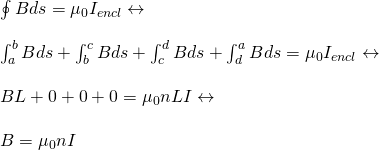where only the "parallel" to the axis "section" inside of the solenoid gives us a non-zero value! By integrating in a length L (a -> b) the integral gives us L, similar to the previous calculations where we had the circumference of a circle. The total enclosed current on the other hand is Iencl = nLI that we will explain in later articles, I guess! :)

### Torodial Solenoid

Let's now consider a donut-shaped torodial soleniod with N turns of wire and carrying a current I. The radius is R. Let's find the magnetic field at all points. We can define 3 circular paths:

1. radius smaller then the donut's (r < R)
2. inside of the donut (r = R)
3. radius larger the the donut's (r > R)

In the paths where r is not equal to R there is no enclosed current, which means that the magnetic field along those paths is zero.

Inside of the donut we have: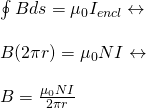which is an equation that looks similar to the straigh-line equation multiplied by N, which is the number of turns!

## REFERENCES:

Mathematical equations that I had in this post where drawn using quicklatex!

### Electric fields:

Getting into Electromagnetism -> electromagnetim, electric charge, conductors, insulators, quantization

Coulomb's law with examples -> Coulomb's law, superposition principle, Coulomb constant, how to solve problems, examples

Electric fields and field lines -> Electric fields, Solving problems around Electric fields and field lines

Electric dipoles -> Electric dipole, torque, potential and field

Electric charge and field Exercises -> examples in electric charges and fields

### Electric flux:

Electric flux and Gauss's law -> Electric flux, Gauss's law

Applications of Gauss's law (part 1) -> applying Gauss's law, Gauss applications

Applications of Gauss's law (part 2) -> more Gauss applications

Electric flux exercises -> examples in electric flux and Gauss's law

### Electric potential:

Electric potential energy -> explanation of work-energy, electric potential energy

Calculating electric potentials -> more stuff about potential energy, potential, calculating potentials

Millikan's Oil Drop Experiment -> Millikan's experiment, electronvolt

Cathode ray tubes explained using electric potential -> cathode ray tube explanation

Electric potential exercises (part 1) -> applications of potential

Electric potential exercises (part 2) -> applications of potential gradient, advanced examples

### Capacitance:

Capacitors (Condensers) and Capacitance -> Capacitors, capacitance, calculating capacitance

How to solve problems around Capacitors -> combination, solving problems, simple example

Electric field energy and density -> Electric field energy, energy density

Dielectric materials -> Dielectrics, dielectric constant, permittivity and strength, how to solve problems

Electric capacitance exercises -> examples in capacitance, energy density and dielectrics

### Current, resistance and EMF:

Electric current -> Electric current, current density

Electrical resistivity and conductivity -> Electrical resistivity, conductivity, thermal coefficient of resistivity, hyperconductivity

Electric resistance -> Resistance, temperature, resistors

Electromotive Force (EMF) and Internal resistance -> Electromotive force, internal resistance

Power and Wattage of Electronic Circuits -> Power in general, power/wattage of electronic circuits

Electric current, resistance and emf exercises -> exampes in all those topics

### Direct current (DC) circuits:

Resistor Combinations -> Resistor combinations, how to solve problems

Kirchhoff's laws with applications -> Kirchhoff's laws, how to solve problems, applications

Electrical measuring instruments -> what are they?, types list, getting into some of them, an application

Electronic circuits with resistors and capacitors (R-C) -> R-C Circuit, charging, time constant, discharging, how to apply

RC circuit exercises -> examples in Kirchhoff, charging, discharging capacitor with/without internal resistance

### Magnetic field and forces:

Magnetic fields -> Magnetism, Magnetic field

Magnetic field lines and Gauss's law of Magnetism -> magnetic field lines, mono- and dipoles, Flux, Gauss's law of magnetism

The motion of charged particles inside of a magnetic field -> straight-line, spiral and helical particle motion

Applications of charged particle motion -> CERN, Cyclotrons, Synchrotrons, Cavity Magetron, Mass Spectrometry and Magnetic lens

Magnetic force applied on Current-Carrying Conductors -> magnetic force on current-carrying conductors/wires, proofs

Magnetic force and torque applied on current loops (circuits) -> magnetic force on current loops, magnetic moment and torque

Explaining the Physics behind Electromotors -> tesla, history and explaining the physics behind them

Magnetic field exercises -> examples in magnetic force, magnetic flux, particle motion and forces/torque on current-carrying conductors

### Magnetic field sources:

Magnetic field of a moving charged particle -> moving charge, magnetic field, force between parallel charged particles

Magnetic field of current-carrying conductors -> magnetic field of current, Biot-Savart law

Force between parallel conductors and the magnetic field of a current loop -> force between parallel conductors, magnetic field of current loop

And this is actually it for today's post! Next time I'm thinking of getting into Magnetic materials, but we might also get into Displacement current first...Either way after these two posts it's Exercise-Time again! :)

Bye!

H2
H3
H4
3 columns
2 columns
1 column